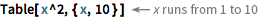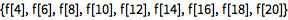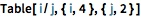The Wolfram Language:Fast Introduction for Programmers

All sectionsGet Started

IteratorsVideo Version

Notes for Java programmers:

Not to be confused with Java's Iterator interface, the Wolfram Language's iterator notation reduces the code required for repetitive operations. Generally, these iterators are used for tasks that would require loops in Java.

Make a table of the first 10 squares:

In:=1`Table[x^2, {x, 10}]`
Out=1Many functions in the Wolfram Language use the standard "iterator specification": min, max, step:

In:=2`Table[f[x], {x, 4, 20, 2}]`
Out=2Notes for Java programmers:

In Java, iterative operations like this would require a for or while loop.

An alternative iterator specification just gives an explicit list of values:

In:=3`Table[f[x], {x, {5, 10, 20, 10, 5}}]`
Out=3Notes for Java programmers:

Using Table this way is analogous to iterating over a Java array or collection with a foreach construct.

Notes for Python programmers:

Using Table in this way is analogous to using the [... for ... in ...] list comprehension construct in Python, though it has somewhat richer semantics.

This makes a nested table:In:=1`Table[i/j,{i,4},{j,2}]`
Out=1Which of these makes a table of the first 20 numbers?

What is the first element of the list produced by Table[f[x], {x, {3, 4, 6}}]?

Which one of these evaluates to the list of squares {1, 4, 9, 16}?